An Integrated Technique Based on Level Set Balloon Force and Laplacian Algorithm for Proficient Analysis of Stem Cell Segmentation | OMICS International
Journal of Molecular Biomarkers & Diagnosis

Like us on:

# An Integrated Technique Based on Level Set Balloon Force and Laplacian Algorithm for Proficient Analysis of Stem Cell Segmentation

1Pondicherry Engineering College, Kalapet, Puducherry-605014, India

2Christ College of Engineering and Technology, Moolakulam Puducherry-605010, India

3Electronics and Communication Engineering, Pondicherry Engineering College, Kalapet, Puducherry-605014, India

*Corresponding Author:
Nathiya R
Research Scholar, Pondicherry Engineering College
Kalapet, Puducherry
Tel:
0413-2654439, 0413-2655256
Fax: 0413- 2655258
E-mail:
[email protected]

Received date: December 30, 2015; Accepted date: January 10, 2016; Published: January 12,2016

Citation: Nathiya R, Priyavadani S, Sivaradje G (2016) An Integrated Technique Based on Level Set Balloon Force and Laplacian Algorithm for Proficient Analysis of Stem Cell Segmentation. J Mol Biomarkers Diagn S8:012. doi:10.4172/2155-9929.S8-012

Copyright: © 2016 Nathiya R, et al. This is an open-access article distributed under the terms of the Creative Commons Attribution License, which permits unrestricted use, distribution, and reproduction in any medium, provided the original author and source are credited

Visit for more related articles at Journal of Molecular Biomarkers & Diagnosis

#### Abstract

Stem cells have impressively great eager in the current time such that it is proved in providing creative new treatments for a vast range of current morbid diseases. The interpretation of stem cells includes segmentation, feature extraction, pattern recognition. This technique leads to analyze the growth rate of stem cells. Segmentation method is proposed to improvice the distance regularized level set evolution with endowing balloon forces. Evolution process in the region which is associated with weaker or without edges that makes the balloon force to control the direction of the evolution which start to slow down the process with this technique laplace operator is included to focus the low contrast images.This method yields segmented images of perfect accuracy because the BDE(Boundary Displacement Error) is decreased and also improved the MDRLS (Modified distance regularized level set) with four well potential achieve better segmentation.

#### Keywords

Mesenchymal stem cells; Modified distance regularized level set; Laplace operator; Balloon force; Boundary displacement error

#### Introduction

Stem cells are undifferentiated biological cells that can differentiate into specialized cells and can divide to produce more stem cells. Mesenchymal stem cells are also called bone marrow stromal cells are multipotent cells that are differentiated into variety of cell types. The segmentation of MSC is proposed to concoct the Modified Distance Regularized Level Set (MDRLS) method. Osher and Sethian first introduced level set method in 1988 . In the precedent two decennium, level set methods have seen the expeditious development in many aspects within the image processing and computer vision field, such as global optimization, etching, deposition, and lithography development and so on , especially in image segmentation. In fact, image segmentation is one of the fundamental tasks in image processing and computer vision. Among the applications in image processing, level set method has great potential for developing image segmentation algorithm. There are large amounts of algorithms and techniques that have been developed to solve image segmentation problems. Malladi et al.,  introduced shape modeling with front propagation based on level set method to implement image segmentation. Caselles et al.,  proposed an implementation to avoid reinitialization procedures in the geodesic active contour (GAC) model. This reinitialization method has been widely used in level set methods [5,6]. Another method for reinitialization is the fast marching algorithm . Although reinitialization as a numerical remedy is able to maintain the regularity of the LSF, it may incorrectly move the zero level set away from the expected position [8,9]. Li et al.,  presented a new variational level set method to force the level set function to close to a signed distance function. Li’s scheme obtained good results for medical image segmentation. Li et al.,  introduced a distance regularized level set evolution (DRLSE) by incorporating a double-well potential function used in the geodesic active contour model . By contrast, the DRLSE is more efficient than conventional level set formulations applying to image segmentation. Ni Aijuan et al.,  proposed an advanced variational formulation based on DRLSE, named ADRLSE (advanced distance regularized level set evolution), that forces the level set function to be close to a signed distance function. The techniques such as thresholding , Fuzzy C-mean and statistical models [14,15], active contour model , watershed segmentation [17,18],and graph cut  have been proposed for medical image segmentation. Gradient Vector Flow (GVF)  snake model is used in image segmentation and this method is proposed to overcome the inability of tracking concavity at boundary. Chuang and Lie  proposed a new downstream algorithm based on Extended Gradient Vector Flow (E-GVF) for segmentation of images.

The main contribution in the proposed method of Mesenchymal stem cell segmentation to revisit the MDRLS model by deploying new balloon forces and laplace operator. The proposed integrated level set method is forceful and ready to effective proper segmentation repercussion in stem cell images. The proposed method is explained in section 2, Section 3 shows the Results and Discussions of this method, Finally concludes this remarks in section 4.

#### Materials and Methods

The main aim of this study is to verify whether the stem cell is healthy or unhealthy. stem cells are the valuable cell source for regenerative medicine. The analysis of stem cell includes segmentation, feature extraction, pattern recognition. The goal of segmentation is to simplify or change the representation of image into multiple segments is proposed to analyze the growth rate of stem cells.The segmentation method is proposed to improvize the distance regularized level set evolution by deploying new balloon forces with this technique laplace operator is included to focus the low contrast images. The initiative function used in distance regularization is single well potential that cause excess backward diffusion and oscillation in LSF, so it is succeeded by double well potential but it losses the capability to conserve the regularity at some points. The diffusion rate is improved in the triple-well potential but the boundary displacement error is increased. This problem is get over by a new method is called four-well potential DRLSE. The segmented output is formed as the final level set function (LSF) by the modified DRLS effect with four minimum points and decreased boundary displacement error. The time lapse images of mesenchymal stem cells is proficiently segmented by this proposed method and the overall block digram shown in given below (Figure 1).

Image filtering

Filtering is one of the most rudimentary operation. It can be applied to reduce the amount of unwanted noise in a particular image. The term filtering can be defined as the value of the filtered image at a given location It can be defined as the value of the separate to refine the image at a given position . The operations of filter can be used to acuminate images, to abolish image noise, to detect and strengthen the edges, or to change the contrast of the image.

Filtering process

The process of filtering includes three different kinds of image enhancement techniques to remove the strong speckle noise in the images. In this method applying 2D adaptive noise removal, median filter and 2D order statistics filter to remove the noise and improve the weak boundaries of the image.The following block diagram explains filtering process.

The 2D Adaptive noise removal filter is a low pass filter and it is used in the gray scale images The median filter is used to remove the salt and pepper noise in the images and the 2D statistics filter is used to remove the speckle noise and to enhance the images (Figure 2).

Figure 2: HPLC chromatogram of the nine reference compounds in 50% aqueous methanol, measured at 370nm. Retention times for rutin, sutherlandin A, sutherlandin B, kaempferol-3-O-rutinoside, sutherlandin C, sutherlandin D, quercitrin, quercetin and kaempferol were 11.9, 12.7, 13.8, 15.3, 16.2, 17.0, 18.0, 26.2 and 28.1 minutes, respectively.

Laplace operator

Laplacian algorithm is used to high spot the images and remove blurring from stem cell images and it is used to make the bright spot becoming much luminous than the encircled pixels in the image. These operator is one of the edge detection operators and simplest sharpening filters, whose response to isolate the pixel is stronger than the response to the edge or line. Laplace operator is a second order differential operator in the n dimensional Euclidean space, and it applied to the image I (x,y) is defined as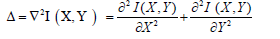=IXX +IYY(1)

Here, I represents an image, X and Y is an input of image, Δ is the Laplace operator. The equation (1) can be enact in distinct form as the following difference equation

? I =(Ii+1,j + Ii-1,j+ Ii,j+1+ Ii,j-1)-4Ii,j ( 2 )

Which illustrates that the gradient of pixel Ii,j only relates to the four adjacent pixels in an image, i.e Ii + 1 j, I i-1 j, Ii,j+1 and Ii j-1,but it is independent of Ii+1 j+1, Ii-1 j-1, Ii-1 j+1 and Ii+1j-1 which are usually on the edge of an image and apply the equilateral direction Laplace operator is defined as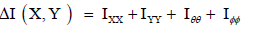(3)

Where X and Y is an input of image, ? is the signed distance function, O is the angle of laplace operator. As the application of Laplace operator, the polished mass becoming much glaring than the walled pixels in the image. So the edge detection function f is easier to become smaller. The line integral of the function and the weighted area of the region are defined by

Lf (?) =∫? g δ (? )| Ñ?|dx( 4 )

Af(?)=∫?fh (-?) dx( 5 )

The energy functional Lf (?) computes the line integral of the function along the zero level contour of ?.The energy functional Af (?) computes a weighted area of region inside zero level set,where δ and H are the dirac delta function and the Heaviside function. For the special case f=1, the energy functional Lf (?) is minimized and AF (?) is exactly the area of the region inside Ω. The function ? minimizes the functional to satisfy the Euler-Lagrange equation.

Distance regularized level set method (DRLS)

The DRLS model provides a effectual narrowband exertion without re-initialization. The edge-based active contour method to drive the level set function (LSF)  to the desiderate boundary.

Energy formulation

Let: φ: Ω→ℜ be a level set function defined on domain Ω. An energy function ε( φ) is defined as: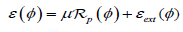(6)

Where >0 is a constant and Rp (φ) is the level set regularization term, defined by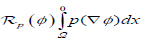(7)

Where p signifies an energy density function p:[0,∞) →ℜ is a potential function, it acts as the penalty term. The energy ε (φ ) ext is designed such that it achieves the zero level set of the LSF is available at desiderate position. The minimization of the energy ε (φ ) can be attained by explaining a level set evolution equation.

native choice of the potential function is p(s) = s2 for the regularization term ?p , which forces ∇φ to be zero. Such a level set regularization term has a steady smoothing effect, but it tends to compress the LSF and make the zero level contour vanish. Such that, the purpose of the level set regularization term is not only to smooth the LSF ?, but also to maintain the signed distance property ∇φ =1, at least in the range of the zero level set, in order to assure an exact estimation for curve

evolution. This method can be done by applying potential p (s) function with a least possible point s=1, such that the level set regularization term (φ ) ?p is minimized when ∇φ =1. Therefore, the potential function should have a minimum point at s=1. The variational level set formulation is evolued by single minimum point. The level set regularization term is referred to as a distance regularization term for its aspect of existence which is the signed distance property of the LSF. An exact definition of the potential for distance regularization is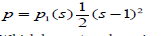(8)

Which has s=1 as the unique minimum point. With this potential p = p1 (s) , the level set regularization term ?p can be explicitly expressed as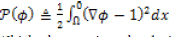(9)

Which characterizes the deviation of φ from a signed distance function. The energy functional is proposed to maintain the signed distance property in the entire process. The level set evolution for energy minimization has an unwanted side effect on the LSF in some crisis. To evade this side effect, precede a new potential function p in the distance regularization term ?p .

The four well potential function is to conserve the signed distance property only in a neighbourhood of the zero level set, although the LSF as a constant, with |∇φ |= 0 , at locations far away from the zero level set.

The four well potential function increase the quality of segmented image with better accuracy. To exact quarter period for a particle in the four-well potential as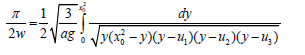(10)

Where w is the frequency, ag denotes the perturbation parameters, x0 is the origin point, u1 is the left well point, u2 and u3 is the right well point and F is the elliptical integral function.

At middle well: Choosing the parameters as, a = u1,b = u2,c = u3, d = x0 2 and then using the equation to find the exact quarter period as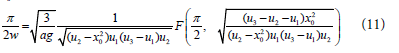At left well: If the particle has negative energy, choose the parameters as a = u1,b = u2,c = u3, d = x0 2 and then using the equation to find exact half period of particle with negative energy as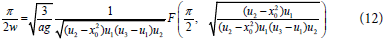At right well 1 and 2: If the particle has positive energy, choose the parameters as as a=u1,b = u2,c = u3, d = x0 2 and then using equation (13,14) to find the exact half period as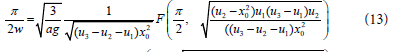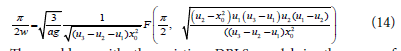The problem with the existing DRLS model in the case of segmentation is that the curve will enduced and bend from the outline in the region with unsustainal or without edges. So the proposed method is used to alter the distance regularization level set method (DRLSM) with four well potential by adding a new balloon force to the process of evolution and debilitate the disentangle contour from ooze at a region with a weak or without an edges.

#### Results and Analysis

(Figure 3) More than 1050 frames of images can be retrieved from the 72 hour video lapse. For the reference only 9 frames of input images have been taken from time lapse video. Stem cells can divide and renew themselves for long periods of time. The mesenchymal stem cells are the multipotent adult progenitor cells. The time lapse video of stem cells can be divided by the frames of images, such that each of the following images have grown under similar circumstances with an estimated time of 8 hours respectively (Figure 4).

The next step is the filtered images of mesenchymal stem cells. Filter is a device or process that removes from a signal from unwanted noises in the images. 2D adaptive noise removal uses a pixel wise adaptive wiener method based on the statistics estimated, mean and standard deviation, from a neighbourhood pixel. Median filtering is used to reduce the noise and preserve edges. The 2D order statistic filter is used to enhance the regions of stem cell images. These filtering replaces each pixel of an image by the nth order element in the sorted set of neighbours of size r by s specified by the nonzero elements in domain.such that progression these filtered images developed under the above conditions with an appraised time of 8 hours sequentialy.

#### Segmentation output

The proposed method of segmentation outputs are simulated for the time lapse images of the mesenchymal stem cell. By using the modified DRLS, the level set evolution of the input image is done and the LSF for the input image are obtained by using the following steps.

Step 1: Parameter setting

Step 2: Smooth image by Gaussian convolution

Step 3: Initialize LSF as binary step function

Step 4: Obtain the initial zero level contour

Step 5: Choose the potential function (four well potential)

Step 6: Start level set evolution

Step 7: Obtain the final zero level contour

Step 8: Display the final zero level contour in mesh to get final level set function.

The above (figure 5) has the segmented images of mesenchymal stem cells, such that each of the following images have grown under similar circumstances with an evaluated time of 8 hours correspondingly. In the MDRLS model, two segmentation steps are applied. The initial step is the developing contour in the direction of the object borderline, when the evolving contour is distant from the object boundary,the process accelerates and when the evolving contour is near to the object boundary, the process slow down.The next step is to observe the clarification of the segmentation results. In each experiment, selected values of ρ, λ and α to be 0.02, 5 and-1 for the first stage and 0.02, 5 and 0 for the second stage, respectively. The zero level set begins as a binary function according to the evolution equation.

Performance evaluation

The modified distance regularized level set segmentation method is evaluated by enumerate the boundary displacement error, PSNR values, Precision, Recall. The Boundary Displacement Error (BDE) measures the average displacement error of one boundary pixels and the closest boundary pixels in the other segmentation. The precision and recall values to characterize the agreement between the oriented boundary edge elements of region boundaries of two segmentations. Comparing to the existing method the PSNR value is increased in four well potential using the second order statistics filter and these filter is used to improve the quality of images compared to the triple well potential and the boundary displacement error is increased in triple well potential so the chances of over segmentation problem arises so it leads to less accuracy. The four well potential is recommended to overcome this problem, These potential function is used to decreased the BDE and to avoid the over segmentation problems and also affirmation of the segmentation can be calculated by precision, recall values (Tables 1 and 2),(Figure 6).

Input Time PSNR Precision Recall Boundary displacement error
Image 1 8 hours 60.8362 99.8350 98.0130 15.2183
Image 2 16 hours 61.5673 99.7350 98.0250 14.5911
Image 3 24 hours 61.7406 99.7017 98.0370 14.4462
Image 4 32 hours 62.2808 99.6850 98.0490 14.0039
Image 5 40 hours 63.1165 99.6750 98.0610 13.3462
Image 6 48 hours 64.7330 99.6683 98.0730 12.1603
Image 7 56 hours 66.0668 99.6636 98.0850 11.2616
Image 8 64 hours 68.8815 99.6600 98.0970 10.7457
Image 9 72 hours 68.2468 99.6572 98.1090 9.9049

Table 1: Evaluation of four well potential.

Input PSNR Precision Recall Boundary displacement error
Input  1 45.1545 81.9800 96.2506 15.9589
Input  2 46.4039 83.9800 95.8177 15.9567
Input  3 46.9154 85.9800 95.3848 15.9551
Input  4 47.1957 87.9800 94.9519 15.9562
Input  5 47.3730 89.9800 94.5190 15.9548
Input  6 47.4953 91.9800 94.0861 15.9543
Input  7 47.5849 93.9800 93.6532 15.9543
Input  8 47.6533 95.9800 93.2203 15.9547

Table 2: Evaluation of triple well potential.

Figure 6: Comparison analysis of (a) PSNR values (b) recall values (c) BDE (d) precision values.

#### Conclusion

In the Proposed method the original DRLS model is integrated by adding a balloon force and the Laplace operator. The most important asset of segmentation method is the ability to design the direction of the evolving contour via different approaches. This model slows down the upcoming contours in regions with hazy edges and dampens the evolving contour from over and above borders of stem cells and also the laplace operator is used to focus the bright spot images becoming much brighter. The quality of segmented image is measured by statistical parameters such as BDE, Precision, Recall get better accuracy. Experimental results have shown that method produces a probable outcome, particularly handle with over-segmentation problems observing with the DRLS model.

#### References

Select your language of interest to view the total content in your interested language

### Article Usage

• Total views: 8782
• [From(publication date):
specialissue-2016 - Dec 11, 2019]
• Breakdown by view type
• HTML page views : 8712Can't read the image? click here to refresh